Get instant live expert help with Excel or Google Sheets“My Excelchat expert helped me in less than 20 minutes, saving me what would have been 5 hours of work!”

#### Post your problem and you’ll get Expert help in seconds.

Your message must be at least 40 characters
Our professional Expert are available now. Your privacy is guaranteed.

# Convert Excel time to decimal hours

Excel has a unique way of storing time through serial numbers, making it possible to perform mathematical operations and conversions to values of time.  This step by step tutorial will assist all levels of Excel users in converting Excel time to decimal hours.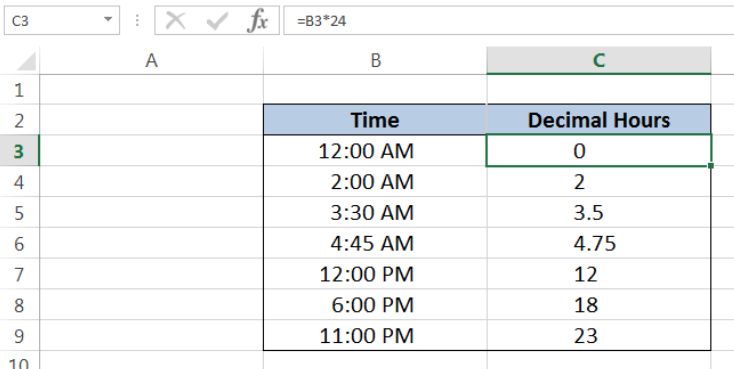Figure 1. Final result: Convert Excel time to decimal hours

## Setting up our Data

Our data consists of two columns: Time (column B) and Result (column C).  We want to convert the time in column B into decimal hours. The results will be recorded in column C.

It is important to set the format for column C to General or Number so that the decimal hours is correctly presented.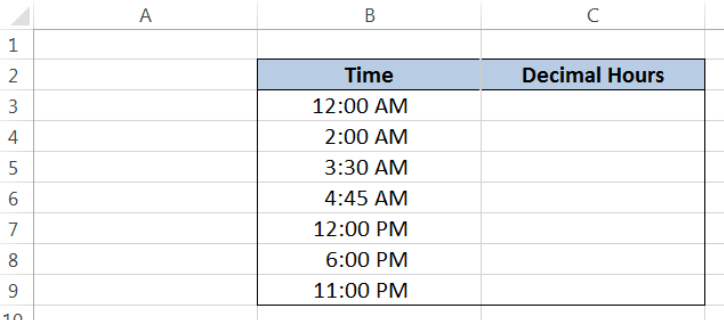Figure 2. Sample data to convert time to decimal hours

## Convert Excel time to decimal hours

Excel time can be easily converted to decimal hours by multiplying the time by 24.  Our formula looks like this:

`Decimal Hours = Time x 24`

## How the formula works

Excel uniquely stores times as numbers.  The value for one whole day is 1. There are 24 hours in one day. Hence, one hour is stored as a fraction of one day or 1/24.

Below table shows some examples of how times are stored in fraction and in decimal form.  The reference time is 12 midnight, which has the value zero “0”.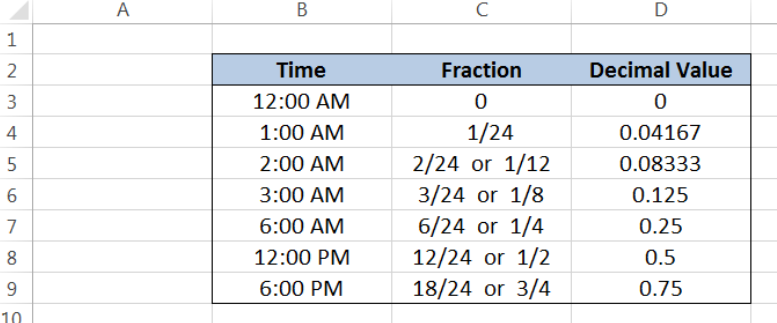Figure 3. Sample data showing how times are stored in Excel

Notice that the value for 6:00 AM is ¼ or 0.25, since it corresponds to one-fourth of one day.  In the same way, 12:00 PM has the value ½ or 0.5, which corresponds to one-half of one day.

Since every hour is represented as 1/24, time can be converted to decimal hours by multiplying it by 24.  This brings us to our formula:

`Decimal Hours = Time x 24`

## Examples:

In order to convert the given times in our data into decimal hours, let us follow these steps:

Step 1.  Select cell C3

Step 2.  Enter the formula: `=B3*24`

Step 3.  Press ENTER

Step 4.  Copy the formula in C3 to cells C4:C9 by clicking the “+” icon at the bottom-right corner of cell C3 and dragging it down

As discussed earlier, the reference for the value of time in Excel is always 12:00 AM, which has a zero value.  Hence, the value in cell C3 is zero.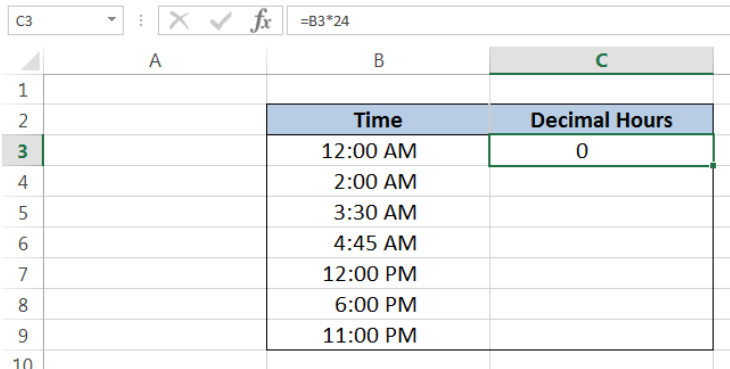Figure 4.  Entering the formula to convert time to decimal hours

In cell C4, we convert 2:00 AM to decimal hours.  2:00 AM has a value of 2/24. When converted to decimal hour, we multiply it by 24.  The formula becomes:

`Decimal hour = 2/24 x 24`

`Decimal hour = 2`

As a result, cell C4 has a value of “2”.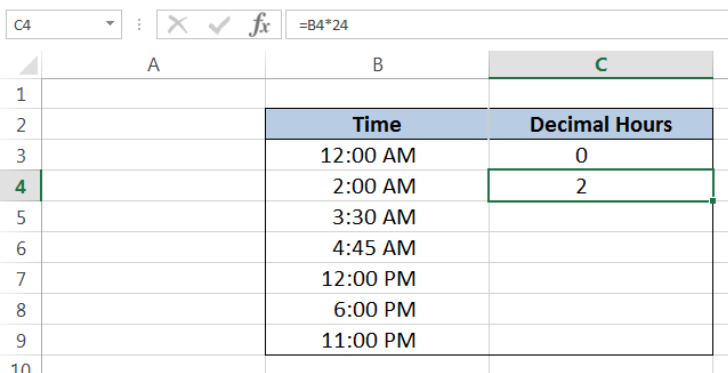Figure 5.  Converting 2:00 AM to decimal hour “2”

The succeeding examples are converted to decimal hours in the same manner.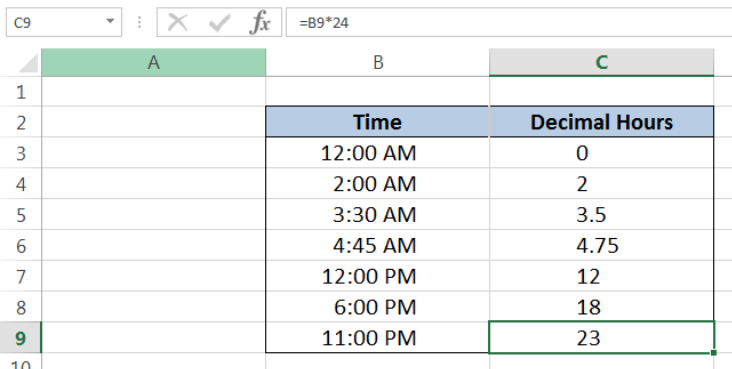Figure 6.  Output: Convert Excel time to decimal hours

## Notes:

• Each succeeding time shows an increasing trend in the value of time from 12:00 AM up to 11:00 PM, as shown above
• 1 hour is equivalent to 60 minutes; hence, 30 minutes is 0.5 of an hour while 45 minutes is 0.75 of an hour

Most of the time, the problem you will need to solve will be more complex than a simple application of a formula or function. If you want to save hours of research and frustration, try our live Excelchat service! Our Excel Experts are available 24/7 to answer any Excel question you may have. We guarantee a connection within 30 seconds and a customized solution within 20 minutes.

### Did this post not answer your question? Get a solution from connecting with the expert.Another blog reader asked this question today on Excelchat:## Subscribe to Excelchat.coAnother blog reader asked this question today on Excelchat: